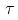NEET  >  Solved Examples: Electromagnetic Induction & Alternating Current

# Solved Examples: Electromagnetic Induction & Alternating Current - Notes | Study NCERT Exemplar & Revision Notes for NEET - NEET

 1 Crore+ students have signed up on EduRev. Have you?

Question 1: A copper disc 20 cm in diameter rotates with an angular velocity of 60 rev s-1 about its axis. The disc is placed in a magnetic field of induction 0.2 T acting parallel to the axis of rotation of the disc. Calculate the magnitude of the e.m.f. induced between the axis of rotation and the rim of the disc.
Solution:-
As the disc rotates, any of its radii cuts the lines of force of magnetic field.
Area swept by radius vector during one revolution
= πr2
= π(10 cm)2
= 100π cm2
= π×10-2 m2
Area swept in one second,
A = (area swept in one revolution) × (Number of revolutions per second)
= π×10-2×60
A = 0.6 πm2
Rate of change of magnetic flux
= dϕB/dt
= BA
= 0.2×0.6π
= 0.12π Wb
According to Faraday’s law, magnitude of induced e.m.f is,
E = dϕB/dt
Therefore, magnitude of e.m.f. is
E = 0.12 πV
Or, E = 0.377 V
Thus from the above observation we conclude that, the magnitude of the e.m.f. induced between the axis of rotation and the rim of the disc would be 0.377 V.

Question 2: A rectangular loop of N turns of area A and resistance R rotates at a uniform angular velocity ω about Y-axis. The loop lies in a uniform magnetic field B in the direction of X-axis. Assuming that at t = 0, the plane of the loop is normal to the lines of force, find an expression for the peak value of the emf and current Induced in the loop. What is the magnitude of torque required on the loop to keep it moving with constant ω?
Solution:-
As ϕ is maximum at t = 0,
ϕ(t) = BA cos ωt
Magnitude of induced emf = N|dϕ/dt|
= BAωN |sinωt|
Magnitude of induced current = [BAωN/R] |sin ωt|
So, peak value of emf = BAωN
peak value of induced current = BAωN/R
To obtain the magnitude of torque required on the loop to keep it moving with constant ω, we have to equate power input is equal to heat dissipation per second.
So, power input = heat dissipation per second
Or,ω = I2R
Or,= [(BAωN)2/R] |sin2ωt|
From the above observation we conclude that,  the magnitude of torque required on the loop to keep it moving with constant ω would be [(BAωN)2/R] |sin2ωt|.

Question 3: An alternating emf 200 virtual volts at 50 Hz is connected to a circuit of resistance 1W and inductance 0.01 H. What is the phase difference between the current and the emf in the circuit. Also find the virtual current in the circuit.
Solution:-
In case of an ac, the voltage leads the current in phase by angle,
ϕ = tan-1 (XL/R)
Here, XL = ωL
= (2πfL)
= (2π) (50) (0.01)
=  πΩ
and
R = 1Ω
So, ϕ = tan-1(π)
≈ 72.3°
Further, irms = Vrms/|Z|
= Vrms/√R2+XL2
Substituting the values we have,
irms = 200/√(1)2+(π)2
= 60.67 amp
From the above observation we conclude that, the virtual current in the circuit would be 60.67 amp.

Question 4: A long solenoid of length 1 m, cross sectional area 10 cm2, having 1000 turns has wound about its centre a small coil of 20 turns. Compute the mutual inductance of the two circuits. What is the emf in the coil when the current in the solenoid changes at the rate of 10 Amp/s?
Solution:-
Let N1 = number of turns in solenoid
N2 = number of turns in coil
Anad A2 be their respective areas of cross-section.
(A1 = A2 in this problem)
Flux ϕ2 through coil crated by current i1 in solenoid is ϕ2 = N2(B1A2)
ϕ= N2 (µ0i1N1/l)A2
Or, ϕ2 = (µ0N1N2A2/l) i2
Comparing with  ϕ2 = Mi1, we get,
Mutual inductance, M = µ0N1N2A2/l
= [4π×10-7×1000×20×10×10-4]/1
= 2.51×10-5 H
So magnitude of induced emf = E2 = M|di1/dt

The document Solved Examples: Electromagnetic Induction & Alternating Current - Notes | Study NCERT Exemplar & Revision Notes for NEET - NEET is a part of the NEET Course NCERT Exemplar & Revision Notes for NEET.
All you need of NEET at this link: NEET

## NCERT Exemplar & Revision Notes for NEET

291 docs
 Use Code STAYHOME200 and get INR 200 additional OFF

## NCERT Exemplar & Revision Notes for NEET

291 docs

### How to Prepare for NEET

Read our guide to prepare for NEET which is created by Toppers & the best Teachers

Track your progress, build streaks, highlight & save important lessons and more!

,

,

,

,

,

,

,

,

,

,

,

,

,

,

,

,

,

,

,

,

,

;